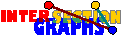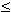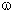# Optimization

Facing an intersection graph model, in applications one often arrives at the problem of computing parameters that are in general hard to compute. When can it be done in polynomial time when restricting to inputs of our intersection graphs?

Clique questions (like computing a maximum clique) are sometimes doable. One reason for this may be the that large octahedron graphs are forbidden induced subgraphs. This maybe caused by Helly property holding for the sets---under certain conditions.

Another frequent issue is that the graphs in question are perfect---then obviously chromatic number, clique number, independence number, and clique covering number can be computed in polynomial time. If Berge`s perfect graph conjecture is true, the only reason for being perfect is the absence of odd cycles of length at least 5 and their complements as induced subgraphs. However it seems difficult to relate perfectness of the intersection class G(P) to properties of the sets P considered. That interval graphs, permutation graphs, unit-interval graphs, ... are perfect doesn`t seem to be caused by intersection properties but rather by the fact that all these graphs are cocomparability graphs, which are perfect. An exception form the chordal graphs.

Since almost every reasonable intersection class contains all complete graphs, there is no hope for having such a class of bounded tree-width. However, in some cases the tree width is a function of the clique number:[S90]: If G is the intersection graph of connected subgraphs of another graph H, then the tree-width w(G) of G is bounded by w(G)w(H)·(G) -1.

Therefore w(G)(G) -1 for chordal graphs, and w(G)(G) -1 for circular-arc graphs. This may serve as an indication towards tractability for such intersection graphs, although the connection is not clear to me.

### Example: Maximum Independent Set

We give a list on what is known (to me) on the problem of finding maximum independent sets for various intersection models:

• Polynomial-time solvable for chordal graphs, and all subclasses, since chordal graphs are perfect.
• Polynomial-time solvable for line graphs. A maximum independent set in L(G) corresponds to a maximum matching in G, note that G can be found in polynomial time.
• NP-complete for unit-disk graphs [CCJ90].
• NP-complete for intersection graphs of straight line segments in the plane, even if all these segments are parallel to three given lines in the plane [KN90].